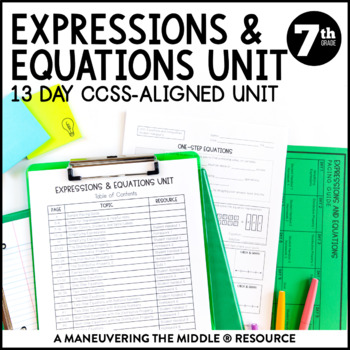digital

# Expressions and Equations Unit: 7th Grade Math (7.EE.1, 7.EE.2, 7.EE.3, 7.EE.4)Subject
Resource Type
Format
Zip (4 MB|100 pages)
Standards
\$13.50
\$13.50The Teacher-Author indicated this resource includes assets from Google Workspace (eg. docs, slides, etc.).

### Description

An 11 day CCSS-Aligned Expressions and Equations Unit includes simplifying expressions, properties of operations, solving one-step equations, and solving two-step equations.

Standards: 7.EE.1, 7.EE.2, 7.EE.3, 7.EE.4

Texas Teacher? Grab the TEKS-Aligned Equations and Inequalities Unit. Please don’t purchase both as there is overlapping content.

1. Unit Overviews

Streamline planning with unit overviews that include essential questions, big ideas, vertical alignment, vocabulary, and common misconceptions. A pacing guide and tips for teaching each topic are included to help you be more efficient in your planning.

2. Student Handouts

Student-friendly guided notes and aligned quick homework that are scaffolded to support student learning. They are available as a PDF and the student handouts/homework/study guides have been converted to Google Slides™ for your convenience.

3. Assessments

A quiz, unit study guide, and editable test allow you to easily assess and meet the needs of your students.

July 2020 Digital Update: All files are available as a PDF, the unit test is editable in PPT, and the student handouts/homework/study guides have been converted to Google Slides™ for your convenience. The Google Slides™ do NOT have text boxes placed, but there is a video tutorial showing you how if you choose.

Please note that classroom activities are NOT included but can be found in my Expressions and Equations Activity Bundle.

Interested in other CCSS-Aligned Expressions and Equations Activities? Check out my Expressions and Equations Activity Bundle, Expressions and Equations Unit Bundle, or 7th Grade Math Curriculum CCSS-Aligned.

Rational Number Operations

Proportional Relationships

Percents

Expressions and Equations

Inequalities

Angle Measures and Triangles

Plane Geometry

Surface Area

Volume

Data and Statistics

Probability

This file is a license for one teacher and their students. Additional licenses are discounted. Please purchase the appropriate number of licenses if you plan to use this resource with your team. Thank you!

Customer Service

Maneuvering the Middle Resources

Maneuvering the Middle on the Web for teacher tips, tricks, and math fun!

Total Pages
100 pages
Included
Teaching Duration
N/A
Report this Resource to TpT
Reported resources will be reviewed by our team. Report this resource to let us know if this resource violates TpT’s content guidelines.

### Standards

to see state-specific standards (only available in the US).
Solve word problems leading to equations of the form 𝘱𝘹 + 𝘲 = 𝘳 and 𝘱(𝘹 + 𝘲) = 𝘳, where 𝘱, 𝘲, and 𝘳 are specific rational numbers. Solve equations of these forms fluently. Compare an algebraic solution to an arithmetic solution, identifying the sequence of the operations used in each approach. For example, the perimeter of a rectangle is 54 cm. Its length is 6 cm. What is its width?
Use variables to represent quantities in a real-world or mathematical problem, and construct simple equations and inequalities to solve problems by reasoning about the quantities.
Solve multi-step real-life and mathematical problems posed with positive and negative rational numbers in any form (whole numbers, fractions, and decimals), using tools strategically. Apply properties of operations to calculate with numbers in any form; convert between forms as appropriate; and assess the reasonableness of answers using mental computation and estimation strategies. For example: If a woman making \$25 an hour gets a 10% raise, she will make an additional 1/10 of her salary an hour, or \$2.50, for a new salary of \$27.50. If you want to place a towel bar 9 3/4 inches long in the center of a door that is 27 1/2 inches wide, you will need to place the bar about 9 inches from each edge; this estimate can be used as a check on the exact computation.
Understand that rewriting an expression in different forms in a problem context can shed light on the problem and how the quantities in it are related. For example, 𝘢 + 0.05𝘢 = 1.05𝘢 means that “increase by 5%” is the same as “multiply by 1.05.”
Apply properties of operations as strategies to add, subtract, factor, and expand linear expressions with rational coefficients.